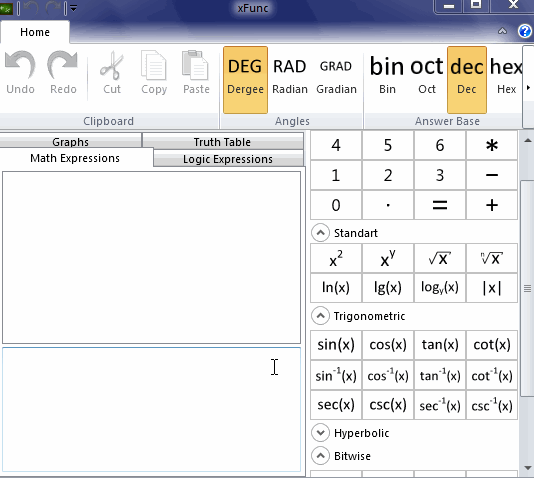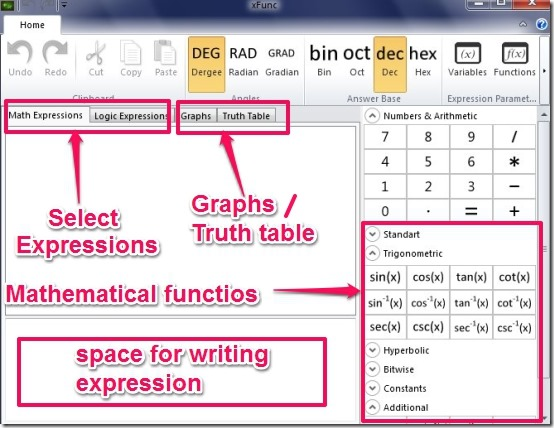Editor Ratings:
User Ratings:
[Total: 1 Average: 5]

Xfunc is free math equation calculator that is used to calculate mathematical expressions. In xfunc you can write complex mathematical equations, evaluate them and then view the answer. Xfunc allows you to use different functions to create any expression. In xfunc you get different sections, that are mathematical expressions, graph, logic and truth table to solve math equation. The answer is calculated very fast and you can calculate multiple mathematical expressions at the same time one by one. I have tested the xfunc on windows 7. You can see below the working of Xfunc to solve math equation.In xfunc you can work with different types of expressions. Below I have shown the working of each of them. See below to get an idea.

## User interface Of Xfunc:

The user interface is really neat and you can use the mathematical functions in the expression, by clicking on functions from the menu at right side. You have 4 different sections in which you can work, which are mathematical expressions, graph, logic and truth table. The different mathematical functions are enough to write almost every mathematical expression. The different mathematical functions you get are:

• Numbers and Arithmetic
• It is used to input numbers, addition, multiplication , division and subtraction.
• Standart
• It includes log, square root, and x raise to the power y and many more.
• Trigonometric
• It includes trigonometric function such as sin, cosine, tan, cosec, cot, sec and inverse of them.
• Hyperbolic
• It includes trigonometric hyperbolic functions.
• Bitwise
• It includes AND, NOT, OR, XOR.
• Constants
• It includes pie and exponent.
• It includes derivative, simplify function, factorial and many more.

You can see below to get an idea of how the user interface looks like.## How To Solve Math Equation:

In order to solve a math equation you have follow the simple steps.

• Run the xfunc.
• Select the type of expression you are working on from the four sections.
• Select the function from the right side menu.
• Enter the value, it can be a number or predefined symbol x or y. Note that only x will work for the expression functions.
• Hit enter and you will have the result.

## Features Of Xfunc:

• Almost every function is covered.
• Clean interface.
• Fast calculation of the math equation.
• No installation required.
• Support for Graphs and Truth Tables.
• Undo option.

You can also try Tibi’s Mathematics Suite. It is free application which allows you to perform some of the major mathematical function such as factorization, matrix calculation, graphing calculator and scientific calculator. So you can try that too.

## Conclusion:

The xFunc is great software for solving math problems. It offers lots of mathematical functions and it can be a great tool for learning purpose. However what I did not like that you cannot save the work, that is, there is no option to save the result to any file.

Overall xfunc is good for calculation and validating the result.